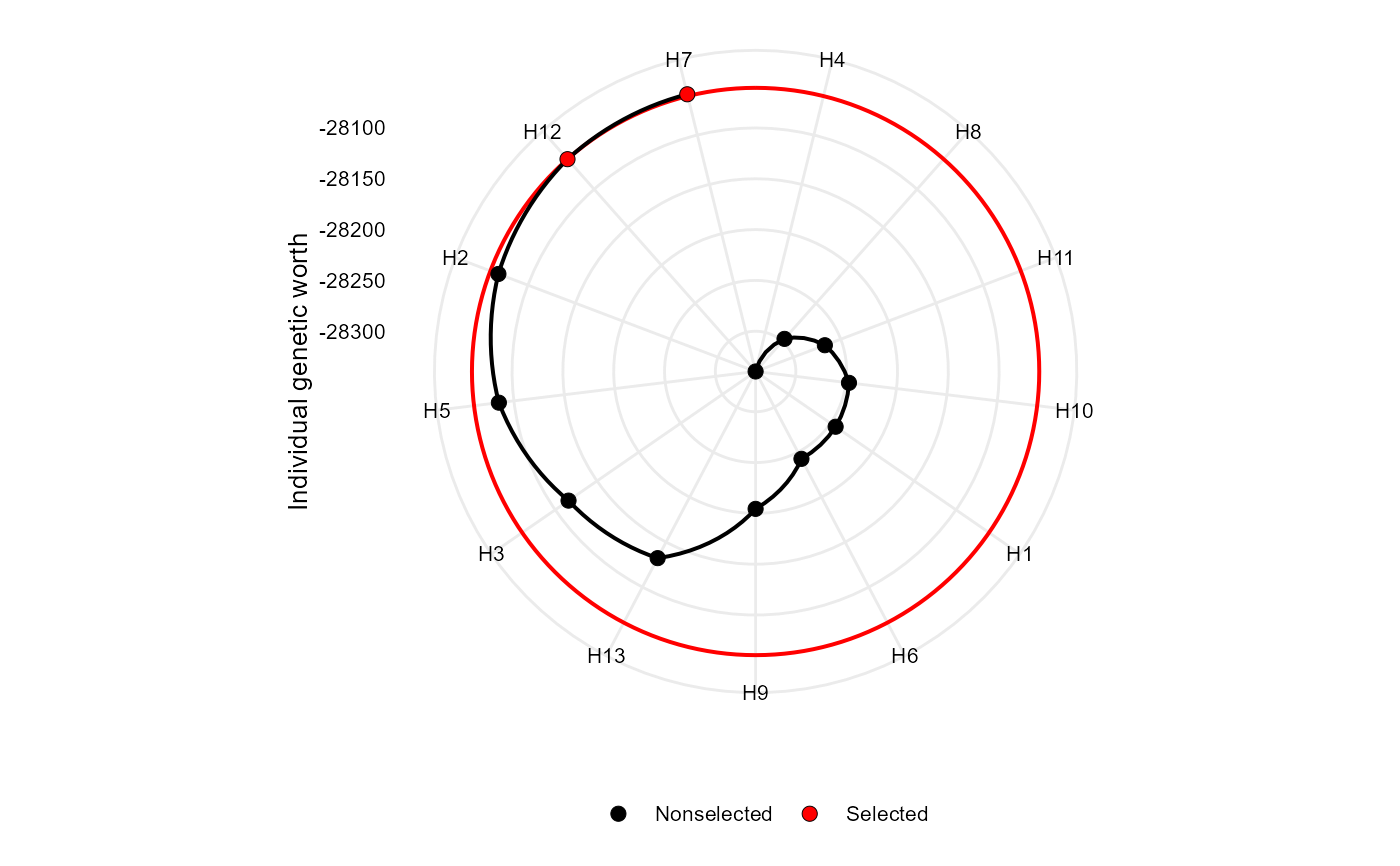Makes a radar plot showing the individual genetic worth for the Smith-Hazel index

## Usage

# S3 method for sh
plot(
x,
SI = 15,
arrange.label = FALSE,
size.point = 2.5,
size.line = 0.7,
size.text = 10,
col.sel = "red",
col.nonsel = "black",
...
)

## Arguments

x

An object of class sh

SI

An integer (0-100). The selection intensity in percentage of the total number of genotypes.

Logical argument. If true (default) a radar plot is generated after using coord_polar().

arrange.label

Logical argument. If TRUE, the labels are arranged to avoid text overlapping. This becomes useful when the number of genotypes is large, say, more than 30.

size.point

The size of the point in graphic. Defaults to 2.5.

size.line

The size of the line in graphic. Defaults to 0.7.

size.text

The size for the text in the plot. Defaults to 10.

col.sel

The colour for selected genotypes. Defaults to "red".

col.nonsel

The colour for nonselected genotypes. Defaults to "black".

...

Other arguments to be passed from ggplot2::theme().

## Value

An object of class gg, ggplot.

## Author

Tiago Olivoto tiagoolivoto@gmail.com

## Examples

# \donttest{
library(metan)
vcov <- covcor_design(data_g, GEN, REP, everything())
means <- as.matrix(vcov$means) pcov <- vcov$phen_cov
gcov <- vcov\$geno_cov

index <- Smith_Hazel(means, pcov = pcov, gcov = gcov, weights = rep(1, 15))
plot(index)# }